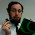Wednesday, July 30, 2014

How I organize statistics

A few discussions at #TMC14 and a recent Twitter conversation with @pamjwilson reminded me how differently I organize the topics in my statistics class.  Instead of trying to explain the what and why of this in 140 characters or less, I made this.

The AP Stats standard syllabus, the golden standard for even non-AP high school statistics classes, organizes the course into four major themes: exploring data (summary stats and graphs), collecting data (sampling and experimental design), anticipating patterns (probability), and inference (confidence intervals and tests).  The groupings make a lot of sense once you have a good idea of how stats works.  They also make a lot of sense if you are efficiently covering procedures through the textbook.  However, I don't think to do a good job introducing the new student into how this collection of procedures can be put to use.

When I completely pulled apart my class and abandoned textbooks, I started with a set of interesting projects first, and then worked backwards to attach relevant topics from the syllabus to the projects.  This took a couple of semesters to iterate, but eventually I noticed a beautiful thing - you could go through a complete flow of collecting, describing, and performing inference on data with every project.  In addition, a natural break between one-variable techniques and two-variable techniques emerged.

Starting with one-variable stats, for the purposes of AP Stats, you can have either quantitative data (numbers) or categorical data (multiple choice options):

• One-variable data describes a population, so you can either perform a census or take a representative sample (SRS or equivalent).
• Once data is collected, it can be summarized (quantitative with center, shape, and spread, and categorical with proportion).
• It can also be graphed (quantitative with histograms, dot plots, and box plots, and categorical with pie graphs and bar graphs).
• If you collected a representative sample and want to know how precisely your data predicts the actual population, you can simulate a sampling distribution and create a confidence interval.
• You can also check that your data provides sufficient evidence to prove a prior hypothesis incorrect by calculating a p-value.
• Finally, I end the cycle by asking students to communicate what they did and what they now know by writing or presenting their process and conclusions.

Now that students have a conceptual overview of collecting, describing, analyzing, and presenting data, we repeat the full cycle with two-variable data.  The first cycle I do is analyzing two quantitative variables:

• Difference in purpose of two-variable analysis (not to describe a population, but to identify relationships between two characteristics/variables within a single population).
• Introduce observational studies.
• Create scatter plots, discuss correlation vs. causation.
• Perform regression and create a model to predict one variable given the other.
• Use a confidence interval or test of the slope to better understand the variability in the model.
I repeat the full cycle one final time with a categorical explanatory variable (group A vs. group B) and either quantitative or categorical response variables.
• Teach experimental design as a way to create two groups that are identical in every way except one key characteristic.
• Compare two sample data graphically (quantitative response with stacked box plots, categorical response with adjacent bar graphs).
• Compare two sample data with confidence intervals and tests to establish that the groups are statistically different (or not).
• End the cycle again with students presenting their conclusions in context.  A statistically significant difference between groups means that the explanatory variable affected the outcome.
If you think that looping this process so many times would require far too much content in the first cycle to effectively teach inference, you are right, unless you teach only the concept and skip the calculation.  I use StatKey, a free, iPad-compatible online simulation calculator, to perform all initial inference calculations with students.  This prevents me from formally having to introduce the normal curve and its probability calculations, lets me skip most of the Central Limit Theorem, and lets me completely ignore the nit-picky assumptions of most intervals and tests.  By the time students get through all of these cycles, they have such a better conceptual foundation for everything we're doing that it is far easier to go back at the end and properly teach probability.  It is simply giving a new technique to calculating numbers they already understand via simulation, but now can understand via the magic of the normal curve and Central Limit Theorem / Binomial Theorem.

If you want a living example of how this organization works, check out my class website.  This fall, I am further modifying it to better use projects to motivate each cycle without having to frontload the content first.  Please comment if you have questions or can push me to think differently about how this is setup.

1 comment:

1.I remember looking at this post a couple days ago and thinking "Well, that's rather brilliant. I don't have any questions". Now with the context that it's apparently different from a standard AP stats course, I'll perhaps at least clarify why I like it.

I like the idea of cycling through multiple times, so that students get to reapply the skills. (Did you go to Mary B & Alex O's session at TMC on spiralling through a curriculum? It feels like that's a bit what you're doing.) Teaching my (Ontario) stats course, I also separate a one variable unit from a two variable unit. (Students still confuse three "quartile" groups with three "median-median" points, but I try.) I tend to start with probability and move into all the data, but I can equally see doing it at the end. So... yeah, good on you for this. I hope the projects plan works out.